# Model Formulation Examples: Goal Programming

In the previous section, we provided the approach to formulate the goal programming model. Here we provide an example of model formulation.

##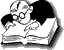Example: Model Formulation

The Japan Life Company produces two products- A and B. According to the past experience, production of either product A or product B requires an average of one hour in the plant. The plant has a normal production capacity of 300 hours a month. The marketing department of the firm reports that because of limited market, the maximum number of product A and product B that can be sold in a month are 140 and 200 respectively. The net profit from the sale of product A and product B are Rs. 600 and Rs. 200 respectively. The manager has set the following goals.

P1: The first goal is to avoid any underutilization of normal production capacity.
P2: He wants to sell maximum possible units of product A and B. Since the net profit from the sale of product A is thrice the amount from Product B, therefore, the manager has thrice as much desire to achieve sales for product A as for Product B.
P3: He wants to minimize the overtime operation of the plant as much as possible.

Solution.

Production capacity

Let x1 = number of units of product A
x2 = number of units of product B

Since overtime operation of the plant is allowed to a certain extent, the constraint can be written as
x1 + x2 + d1  - d1+ = 300

Where
d1 = underutilization (idle) of production capacity and
d1+ = overtime operation of the normal production capacity.

Sales constraints

In this case, the maximum sales for product A and product B are set at 140 and 200 respectively. Hence, it is assumed that overachievement of sales beyond the maximum limits is impossible. Then, the sales (market) constraints can be expressed as

x1 + d2 = 140
x2 + d3 = 200

Where d2 and d3 are the underachievement of the sales goal for product A and B respectively.

Therefore, the complete goal programming model can be written as

Minimize z = P1d1 + 3P2d2 + P2d3 + P3d1+

subject to

x1 + x2 + d1  - d1+ = 300
x1 + d2 = 140
x2 + d3 = 200

and x1, x2, d1, d2, d3, d1+ ≥ 0

Share and Recommend

•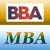MBA & BBA
•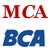BCA & MCA
•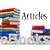Ebooks & Articles
•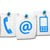Contact us